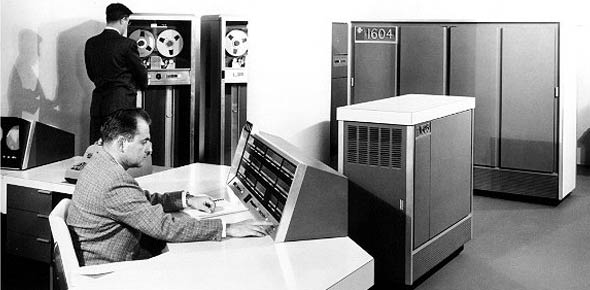# Volume 2 CDC 2A651 ( Q

15 Questions | Total Attempts: 186SettingsThis is awsome practice!

• 1.
An unbalanced force on a body produces  or tends to produce an acceleration in the direction of the force and that the acceleration, if any, is directly proportional to the force and inversely proportional to the mass pf the body. " this statement is
• A.

Bernoulli's principle.

• B.

Newton's first law of motion.

• C.

Newton's second law of motion.

• D.

Newton's third law of motion

• 2.
Which type of duct would decrease the velocity and increase the pressure of a gas as it passes through?
• A.

Elbow.

• B.

Straight.

• C.

Divergent.

• D.

Convergent.

• 3.
"The combination of decreased pressure above an airfoil, and the increased pressure below the airfoil produces lift" is best described by
• A.

Bernoulli's principle.

• B.

Newton's first law of motion

• C.

Newton's second law of motion

• D.

Newton's third law of motion

• 4.
The tendency of an object at rest to remain at rest and the tendency of an object in motion to continue its motion in a straight line at the same speed is called
• A.

Energy.

• B.

Inertia.

• C.

Friction

• D.

Potential.

• 5.
Which section of a jet engine introduces and burns fuel?
• A.

Turbine.

• B.

Diffuser.

• C.

Compressor.

• D.

Combustion.

• 6.
Which section of a jet engine keeps the compressor rotating?
• A.

Turbine.

• B.

Exhaust.

• C.

Diffuser

• D.

Combustion.

• 7.
Which method of producing thrust does a turboprop engine use?
• A.

Accelerating a large mass of air by a small amount.

• B.

Moving a small quantity of air through a large velocity change

• C.

Using the same working fluid for propulsive force as that used within the engine.

• 8.
Which type of horsepower is delivered to the propeller for useful work?
• A.

Brake.

• B.

Indicated.

• C.

Frictional.

• D.

Equivalent shaft.

• 9.
The heat content per pound of kerosene is how many Btu's?
• A.

10,500

• B.

15,000

• C.

18,500

• D.

40,000

• 10.
To measure barometric pressure, you would use a
• A.

Potentiometer.

• B.

Psychrometer

• C.

Pyrometer.

• D.

Barometer.

• 11.
What is the sea-level atmospheric pressure at "standard day" conditions?
• A.

13.5 psi

• B.

14.7 psi

• C.

49.94

• D.

40.55 psi

• 12.
What effect does a divergent design of a diffuser have on air pressure?
• A.

Increases pressure.

• B.

Decreases pressure.

• C.

Has no effect on pressure

• D.

Results in pressure fluctuations.

• 13.
Which engine component meters fuel for combustion?
• A.

P&D valve.

• B.

Fuel Pump.

• C.

Fuel control.

• D.

Fuel nozzles.

• 14.
What directs the gases onto the first-stage turbine wheel blades in a jet engine?
• A.

Flameholder.

• B.

Combustion chamber.

• C.

• D.

Turbine stator.

• 15.
When two or more turbine wheels are used in a jet engine,which component
• A.

Diffuser

• B.

Jet nozzle.

• C.

Combustion chamber

• D.

Turbine stator

Related TopicsBack to top